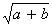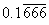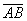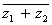## Definition Of Vinculum

Vinculum is a horizontal bar that is drawn on a mathematical expression to indicate that they form a group.

Vinculum is a Latin word that means 'bond' or 'tie'.

### Examples of Vinculum

The horizontal bar used in the given examples is vinculumA radical --For repeating decimals --Line segment AB --Complex conjugate --### Solved Example on Vinculum

#### Ques: Which of the following is the correct vinculum sign used to represent the square root of the sum of the two numbers a and b?

##### Choices:Step 2: The vinculum form of the sum of the square root of the two numbers a and b is